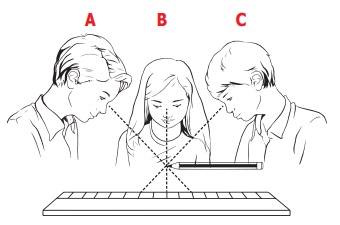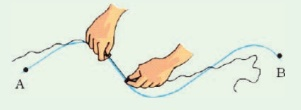Home | | Science 6th Std | Length

# Length

What is length? The distance between one end and the other desired end is called as length. It may be the distance between the edges of a book or a table or the corners of a football ground or even from your home to school.

Length

What is length? The distance between one end and the other desired end is called as length. It may be the distance between the edges of a book or a table or the corners of a football ground or even from your home to school.The standard unit of length is 'Metre'. It is represented by letter ‘m’. Very small lengths can be measured in mm and cm. Still larger measures, say height of a building, a banner or a lamp post are all measured in metre. How to express still longer lengths? Say distance between two cities or villages or distance between your school and home? It is expressed in kilometre (km).

Know the unit of length

1 km (kilometre) = 1000 m (metre)

1 m (metre) = 100 cm(centimetre)

1 cm (centimetre) = 10 mm (millimetre)

Think: Can you convert 1 km in terms of cm?Measure the length of your pencil now. For sure the lengths of all your pencils are not the same.

1. Take the meter scale

2. Check lines with marking 1,2,3,4 ... till 15 (for smaller scales) or till 30 (bigger scales). The distance between 1 and 2 is denotes a centimetre (it is written as ‘cm’).

3. Notice, in between 1 and 2 there will be smaller markings. If you count, there will be 9 such lines. The distance between any two consecutive smaller markings within a ‘cm’ denotes a millimetre (written as ‘mm’).

Let us now understand more about the three important quantities – Length, Mass and Time.

From the activity:1 you see that your measurement is different from that of your friends. Also different measuring units are used in different countries.

Activity 1Form a group of 5 members. Select one person and others measure her/his height individually using your hand span and cubit. Compare your answers with others. Do you find any differences? Why? Now you all stand in front of a wall and mark your height on the wall. Measure your height with a scale. What differences do you infer?

Why do we need SI Units?

The sake of uniformity, scientists all over the world have adopted a common set of units to express measurements. This system is called as the International System of Units or SI Units.

SI unit for length is Metre

SI Unit for mass is Kilogram

SI Unit for time is Second

Area of Surface = m2

Volume of Solid = m3

Multiples and sub multiples of SI Units

Prefixes used in SI Units. (Always remember the base unit is metre, litre, kilogram)

Corrective Measures for Measurement

Measurement has to be accurate and the approach has to be correct always. In our day to day life approximation may not have much impact. But it has a large impact in scientific calculations. For example, if the curvature of key (lock and key) is changed by even 1 mm, the lock would not open. Let us look at some common mistakes that occur while using a scale.

To measure the length of a pin using a scale* The head of the pin has to coincide with ‘0’ of the scale.

* Count the number of centimetre and from there count the number of finer divisions. The count of the division is ‘mm’

* In the above example the length of pin is 2 cm and 6 mm.In the given activity, measure the quantities using suitable measuring units and express them with suitable multiple and submultiples.* Take care to write the correct submultipleParallax is a displacement or difference in the apparent position of an object viewed along two different lines of sight.Correct position of the eye is also important for taking measurement. Your eye must be exactly in front of vertically above the point where the measurement has to be taken. In the above representation, to avoid parallax error, reading will be correct. From positions ‘A’ and ‘C’, the readings will be different and erroneous.

Methods of measuring Length of a curved line:

Activity 2

Aim: To find the length of a curved line using a string.

Materials needed: A meter scale, a measuring tape, a string and a sketch pen

Method:

* Draw a curved line AB on a piece of paper

* Place a string along the curved line. Make sure that the string covers every bit of the curved line.

* Mark the points where the curved line begins and ends on the string.

* Now, stretch the string along the length of a meter scale and measure the distance between the two markings of the string. Note it.

* This will give you the length of a curved line.Find the length of a banana.Activity 3

Measuring the length of a curved line using a dividerDraw a curved line AB on a piece of paper

Separate the legs of the divider by 0.5 cm or 1 cm using a ruler.

Place it on the curved line starting from one end. Mark the position of the other end. Move it along the line again and again cutting the line into number of segments of equal lengths. The remaining parts of the line can be measured using a scale.

Count the number of segments

Therefore, the length of the line = (number of segments × length of each segment) + length of the left over part.Tags : Measurements | Term 1 Unit 1 | 6th Science , 6th Science : Term 1 Unit 1 : Measurements
Study Material, Lecturing Notes, Assignment, Reference, Wiki description explanation, brief detail
6th Science : Term 1 Unit 1 : Measurements : Length | Measurements | Term 1 Unit 1 | 6th Science